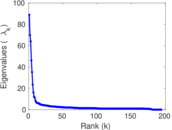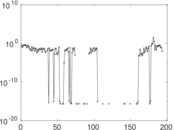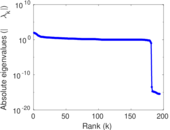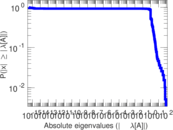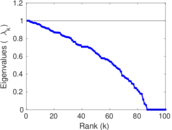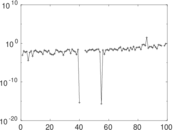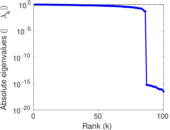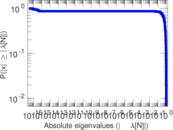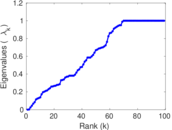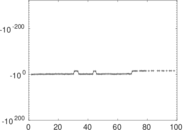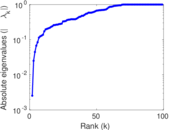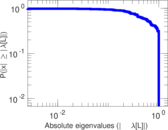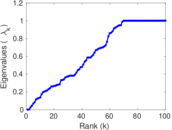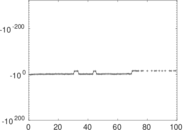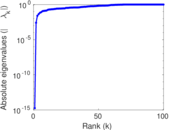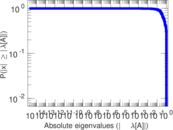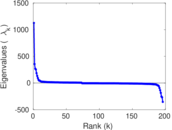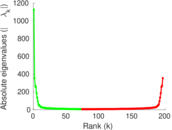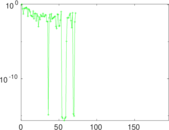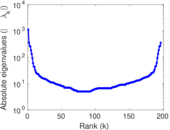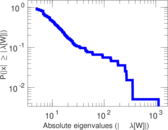# Wikibooks edits (bs)

This is the bipartite edit network of the Bosnian Wikibooks. It contains users and pages from the Bosnian Wikibooks, connected by edit events. Each edge represents an edit. The dataset includes the timestamp of each edit.

 Code `bbs` Internal name `edit-bswikibooks` Name Wikibooks edits (bs) Data source http://dumps.wikimedia.org/ AvailabilityDataset is available for download Consistency checkDataset passed all tests Category Authorship network Dataset timestamp 2017-10-20 Node meaning User, article Edge meaning Edit Network formatBipartite, undirected Edge typeUnweighted, multiple edges Temporal dataEdges are annotated with timestamps

## Statistics

 Size n = 1,135 Left size n1 = 196 Right size n2 = 939 Volume m = 2,572 Unique edge count m̿ = 1,447 Wedge count s = 98,740 Claw count z = 7,676,736 Cross count x = 490,371,652 Square count q = 29,438 4-Tour count T4 = 635,494 Maximum degree dmax = 529 Maximum left degree d1max = 529 Maximum right degree d2max = 134 Average degree d = 4.532 16 Average left degree d1 = 13.122 4 Average right degree d2 = 2.739 08 Fill p = 0.007 862 25 Average edge multiplicity m̃ = 1.777 47 Size of LCC N = 879 Diameter δ = 16 50-Percentile effective diameter δ0.5 = 5.320 53 90-Percentile effective diameter δ0.9 = 9.734 16 Median distance δM = 6 Mean distance δm = 5.884 32 Gini coefficient G = 0.707 413 Balanced inequality ratio P = 0.227 061 Left balanced inequality ratio P1 = 0.131 026 Right balanced inequality ratio P2 = 0.320 373 Relative edge distribution entropy Her = 0.814 542 Power law exponent γ = 3.511 62 Tail power law exponent γt = 3.191 00 Tail power law exponent with p γ3 = 3.191 00 p-value p = 0.000 00 Left tail power law exponent with p γ3,1 = 1.881 00 Left p-value p1 = 0.517 000 Right tail power law exponent with p γ3,2 = 4.331 00 Right p-value p2 = 0.138 000 Degree assortativity ρ = −0.133 225 Degree assortativity p-value pρ = 3.654 56 × 10−7 Spectral norm α = 88.873 1 Algebraic connectivity a = 0.002 539 43 Spectral separation |λ1[A] / λ2[A]| = 1.272 32 Controllability C = 759 Relative controllability Cr = 0.677 074

## Plots

### Fruchterman–Reingold graph drawing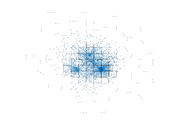### Degree distribution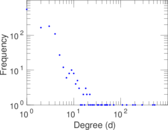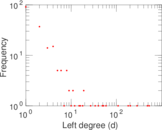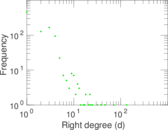### Cumulative degree distribution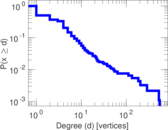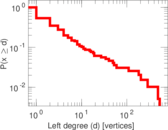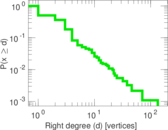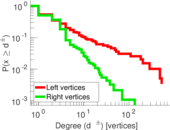### Lorenz curve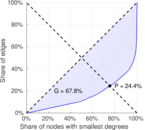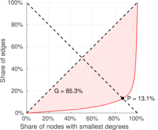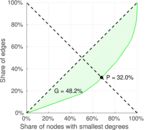### Spectral distribution of the adjacency matrix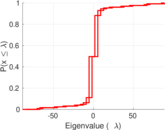### Spectral distribution of the normalized adjacency matrix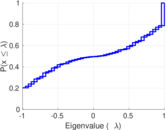### Spectral distribution of the Laplacian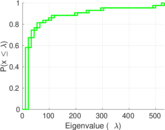### Spectral graph drawing based on the adjacency matrix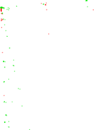### Spectral graph drawing based on the Laplacian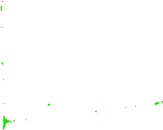### Spectral graph drawing based on the normalized adjacency matrix### Degree assortativity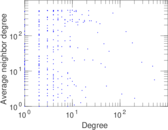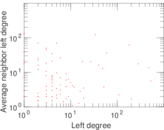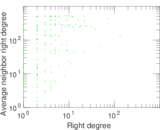### Zipf plot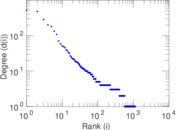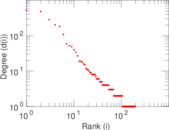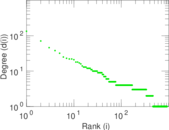### Hop distribution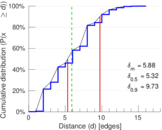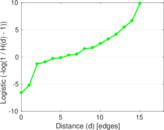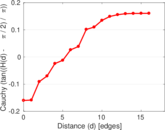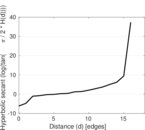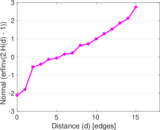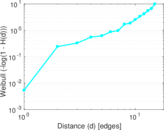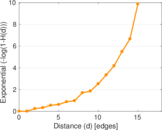### Double Laplacian graph drawing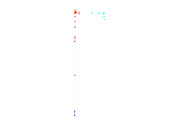### Delaunay graph drawing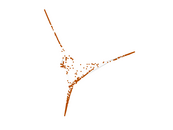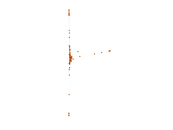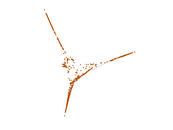### Edge weight/multiplicity distribution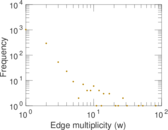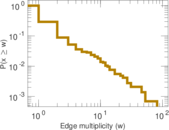### Temporal distribution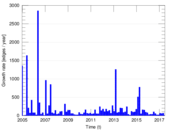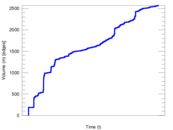### Temporal hop distribution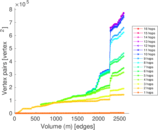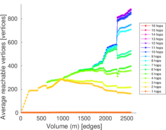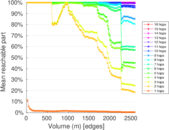### Diameter/density evolution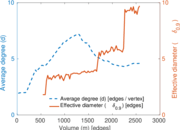### Matrix decompositions plots